# Lens equation – Concave lens and examples

The lens equation is:

1/F=1/(do)+1/(di)

Where

 F = Principal focal length do = Object distance di = Image distance

But to continue, we need to be careful and clear in our understanding of what is negative and what is positive.

## Concave single lens

In all cases and situations of the concave lens the image will always be.

SMALLER

UPRIGHT

VIRTUAL (cannot be projected on a screen)

Between principal focal point and the concave lens

and to this list we should now add: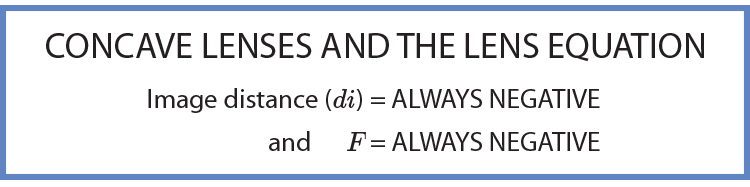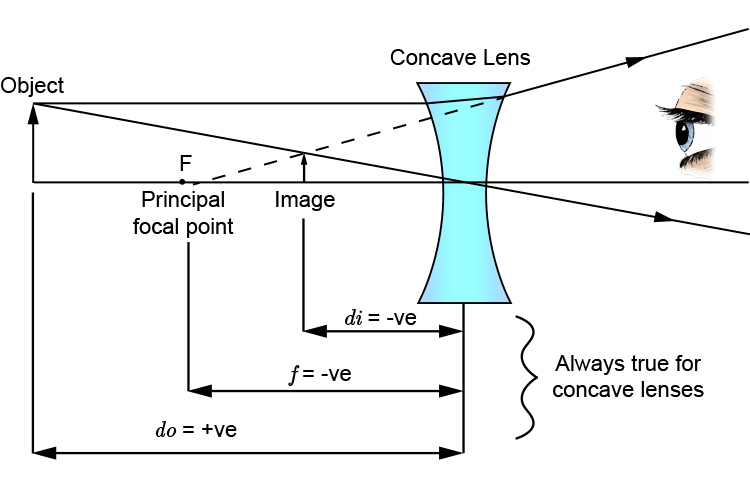Examples

Question 1

If an object is 12cm from a concave lens with a focal length of -6cm what is the image distance from the lens and is the image upright or upside down?

Immediately we see a minus principal focal length (we presume this is what the person who set the exam paper means by just saying focal length) then we know we must be dealing with a concave lens.

Knowing we are dealing with a concave lens we know the image will be upright.

Draw a quick sketch to remind ourselves: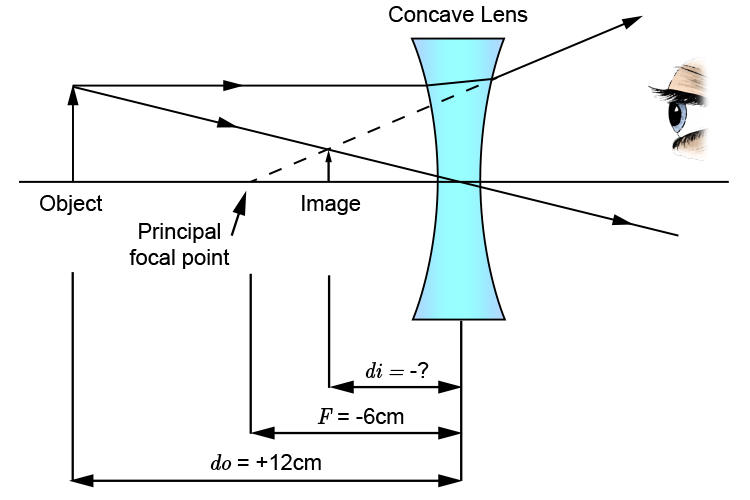From this quick reminder we have

1/F=1/(do)+1/(di)

1/-6=1/12+1/(di)

-0.16dot6=0.083dot3+1/(di)

1/(di)=-0.25

Multiply both sides by di and divide both sides by -0.25 to get di on its own.

1/-0.25=di

di=-4

and looking at the sketch we know all concave images are upright.

Answer = -4 and UPRIGHT

Question 2

A concave lens of focal length 15cm forms an image 10cm from the lens. How far is the object from the lens?

As we are given the fact that this is a concave lens we should draw a quick sketch to remind ourselves.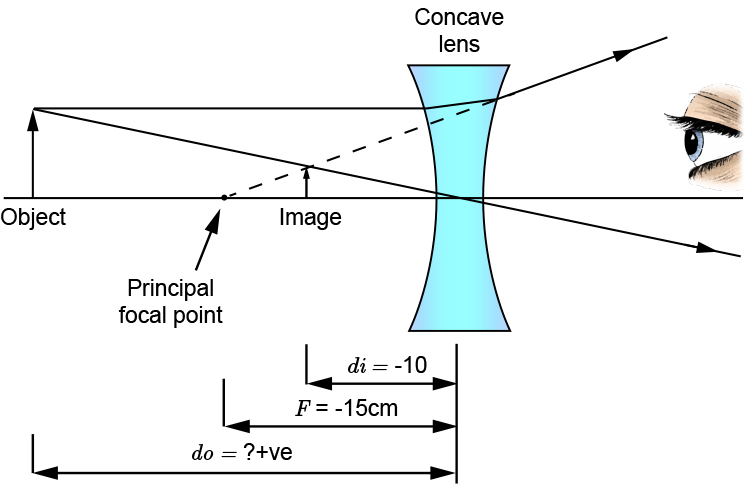We have to assume two things

i.  When focal length of 15cm is mentioned we must assume that this means principal focal length and being a concave lens the focal length must be -15cm

ii. Although an image is formed at 10cm because this is a concave lens this must be -10cm

From this quick sketch we can follow with the lens equation which gives us.

1/F=1/(do)+1/(di)

1/-15=1/(do)+1/-10

-0.066dot6=1/(do)-0.1

1/(do)=-0.066dot6+0.1

1/(do)=0.0333

Multiply both sides by do and divide both sides by 0.0333 to get do on its own.

1/0.0333=do

do=30cm

Example 3

A nail is placed a distance of 40cm from a diverging lens and an image is produced at a distance of 10.91cm from the lens. What is the distance of the principal focal point from the lens?

As soon as we can, when we read that there is a diverging lens we should draw a quick sketch to remind ourselves of the details of this lens: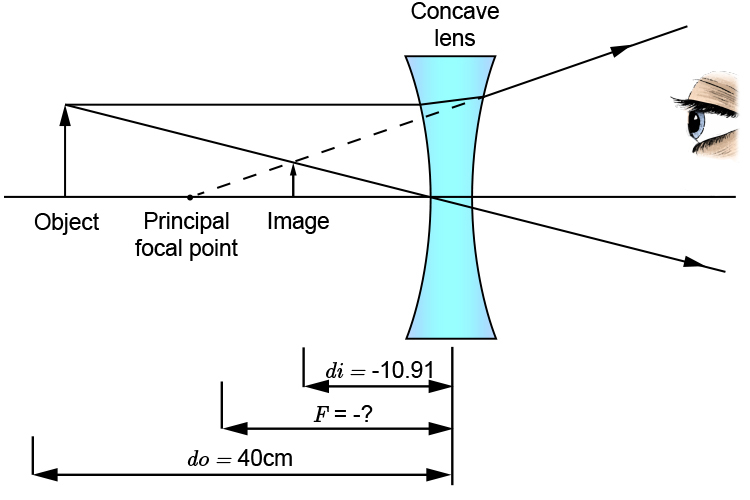We should realise that the image distance is not just 10.91cm but minus 10.91cm. We should also realise that the answer we are seeking should provide us with a minus number for the focal point.

Now we should recall the lens formula:

1/F=1/(do)+1/(di)

1/F=1/40+1/-10.91

1/F=0.025-0.091659

1/F=-0.066659

Multiply both sides by F and divide both sides by -0.66659 we get.

F=1/-0.066659

F=-15

As we hoped, we get a minus principal focal length of -15